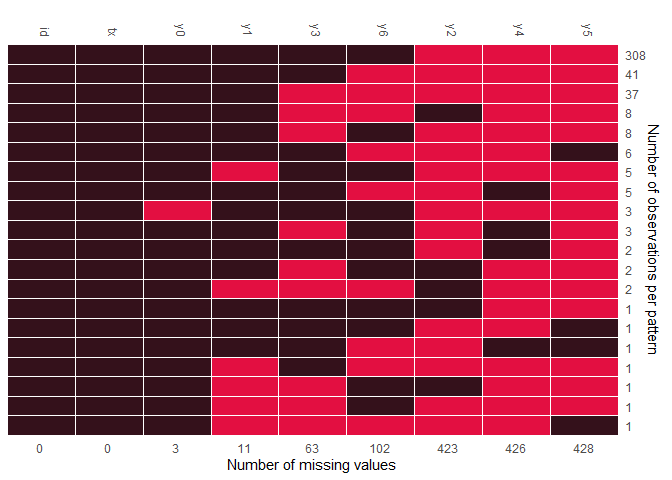## Introduction to remiod

remiod is acronymed from REference-based Multiple Imputation of Ordinal or Binary variables (Wang and Liu 2022). The purpose of the package is to facilitate the implementation of sensitivity analyses to ordinal/binary response variable with missingness.

Missing data are common in randomised controlled trials (RCTs) and can bias results if not handled appropriately. A statistically valid analysis under the primary missing-data assumptions, usually missing at random (MAR), can be planned, followed by sensitivity analyses under alternative justified assumptions to assess the robustness of results. Reference-based Multiple Imputation (MI) and delta adjustment procedures thus have been developed for analysis under missing-not-at-random (MNAR) assumptions.Tools in this package are developed specifically for reference-based analyses of binary or ordinal responses.

In this vignette, we use the Schizophrenia data which is a part of the remiod package. For more info on this data set, check the help file or visit the following link. The original data is in a long format from a clinical trial, i.e. one row per patient per visit. We first reshape it into a wide format.

``````data(schizo)

schizow = dcast(schizo, id + tx ~ week, value.var = "imps79o")
colnames(schizow) = c(colnames(schizow)[1:2], paste0("y",colnames(schizow)[-c(1:2)]))
schizow = as.data.frame(schizow)
schizow[,colnames(schizow)[-c(1:2)]] = lapply(schizow[,colnames(schizow)[-c(1:2)]],
function(x) factor(x, levels = c("1", "2", "3", "4"), ordered=TRUE))

head(schizow) %>% kbl(align = "c") %>%
kable_classic(full_width = F, html_font = "Cambria") %>%
column_spec(1:2, width = "2cm") %>%
add_header_above(c(" " = 1," "=1, "Responses at the baseline, week 1- week 6" = 7))
``````
Responses at the baseline, week 1- week 6
id tx y0 y1 y2 y3 y4 y5 y6
1103 1 4 2 NA 2 NA NA 2
1104 1 4 2 NA 1 NA NA 2
1105 1 2 2 NA 1 NA NA NA
1106 1 2 1 NA 1 NA NA 1
1107 0 3 3 NA 3 NA NA 4
1108 1 4 4 NA 2 NA NA 3

`tx` is the treatment variable with 1 being treated and 0 being placebo. `y0`,`y1`,…,`y6` are the response variables from baseline (visit=0) to visit 6. First, we check the missing pattern of variables in this data using function `md_pattern` from `JointAI`.Figure 1. Missing pattern of Schizophrenia data

Figure 1 indicates there were missing values on the response variable at each visit from the baseline to the last visit. Applying sequential modeling method described by Tang (Tang 2018), `remiod` function can be set up as follows:

``````test = remiod(formula = y6 ~ tx + y0 + y1 + y2 + y3 + y4 + y5, data=schizow,
trtvar = 'tx', algorithm = "jags", method = "MAR",
n.iter = 0, warn = FALSE, mess = FALSE)
list.models(test)

>   Order model_formula
> 1 1     y0 ~ tx
> 2 2     y1 ~ tx + y0
> 3 3     y3 ~ tx + y0 + y1
> 4 4     y2 ~ tx + y0 + y1 + y3
> 5 5     y4 ~ tx + y0 + y1 + y2 + y3
> 6 6     y5 ~ tx + y0 + y1 + y2 + y3 + y4
> 7 7     y6 ~ tx + y0 + y1 + y2 + y3 + y4 + y5
``````

In the default setting, the sequence of models are ordered based on the number of missingness on each variables, which leads to the model on `y3` (the number of missing values is 63 as showed in Figure 1) preceding to the model on `y2` (the number of missing values is 423 as showed in Figure 1). In case the sequence of models is expected to follow time order of visits, option `model_order = paste0("y",0:5)` can be added to specify the model order as `y0`, `y1`, `y2`, `y3`, `y4`, and `y5`, for example:

``````test_ord = remiod(formula = y6 ~ tx + y0 + y1 + y2 + y3 + y4 + y5, data=schizow,
trtvar = 'tx', algorithm = "jags", model_order = paste0("y",0:5),
method = "MAR", n.iter = 0, warn = FALSE, mess = FALSE)
list.models(test_ord)

>   Order model_formula
> 1 1     y0 ~ tx
> 2 2     y1 ~ tx + y0
> 3 3     y2 ~ tx + y0 + y1
> 4 4     y3 ~ tx + y0 + y1 + y2
> 5 5     y4 ~ tx + y0 + y1 + y2 + y3
> 6 6     y5 ~ tx + y0 + y1 + y2 + y3 + y4
> 7 7     y6 ~ tx + y0 + y1 + y2 + y3 + y4 + y5
``````

Argument `method` in `remiod` function is the place to request controlled multiple imputation. `method = "MAR"` in the example setting above will give imputation based on MAR assumption. Other options include `J2R` (Jump to Reference), `CR` (Copy Reference), and `delta` (delta adjustment).Theoretic aspects of these methods can be found in Tang (2018).

``````test_mar = remiod(formula = y6 ~ tx + y0 + y1 + y3, data = schizow, trtvar = 'tx',
algorithm = "jags", method = "MAR", ord_cov_dummy = FALSE,
n.iter = 2000, n.adapt = 2000, n.chains = 2, warn = FALSE, mess = FALSE)
``````

Function `extract_MIdata` can be used to extract an expected number, say `M = 10`, of imputed data sets following MCMC sampling of `remiod`. Argument `minspace` sets the distance, i.e. the number of iterations, between two subsequently selected MCMC samples. If post-processing MCMC samples is required, it can be set through argument `mi.setting`. For example, `mi.setting = list(exclude_chains=2)` requests to exclude the second chain of MCMC samples.

``````extdt = extract_MIdata(object=test_mar, method="J2R",mi.setting=NULL, M=10, minspace=2)
table(extdt[]\$Imp_)
>
>   1   2   3   4   5   6   7   8   9  10
> 437 437 437 437 437 437 437 437 437 437
``````

## References

Tang 2018. “Controlled Pattern Imputation for Sensitivity Analysis of Longitudinal Binary and Ordinal Outcomes with Nonignorable Dropout.” Statistics in Medicine 37 (9): 1467–81.

Wang and Liu. 2022. “Remiod: Reference-Based Controlled Multiple Imputation of Longitudinal Binary and Ordinal Outcomes with Non-Ignorable Missingness.” arXiv 2203.02771.